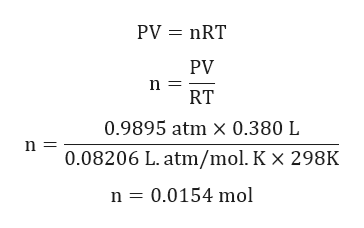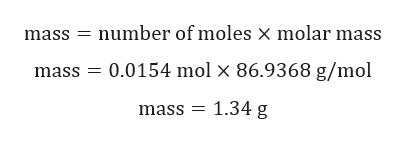# Based upon the following balanced equation:MnO2(s)  +  4 HCl(g)  →  MnCl2(aq)  +  2 H2O(l)  +  Cl2(g)How many grams of manganese(IV) oxide (MnO2) should be added to an excess of hydrogen chloride gas to obtain 380 mL of chlorine gas at 25oC and 752 mmHg?Assume you can ignore the vapor pressure of water.Your answer should have 3 significant figures.  Do not include the units in your answer.

Question
3 views

Based upon the following balanced equation:

MnO2(s)  +  4 HCl(g)  →  MnCl2(aq)  +  2 H2O(l)  +  Cl2(g)

How many grams of manganese(IV) oxide (MnO2) should be added to an excess of hydrogen chloride gas to obtain 380 mL of chlorine gas at 25oC and 752 mmHg?

Assume you can ignore the vapor pressure of water.

check_circle

Step 1

Given:

Volume = 380 mL = 0.380 L.

Temperature = 25oC = 298 K

Pressure = 752 mmHg = 0.9895 atm.

MnO2(s)  +  4 HCl(g)  →  MnCl2(aq)  +  2 H2O(l)  +  Cl2(g)

Step 2

Calculation for number of moles of Cl2:help_outlineImage TranscriptionclosePV nRT PV RT 0.9895 atm X 0.380 L n 0.08206 L. atm/mol. K x 298K n 0.0154 mol fullscreen
Step 3

Calculation for mass of MnO2:

1 mole of MnO2 gives 1 mole of Cl2.

0.0154 mole of MnO...help_outlineImage Transcriptionclosenumber of moles x molar mass mass mass 0.0154 mol x 86.9368 g/mol mass 1.34 g fullscreen

### Want to see the full answer?

See Solution

#### Want to see this answer and more?

Solutions are written by subject experts who are available 24/7. Questions are typically answered within 1 hour.*

See Solution
*Response times may vary by subject and question.
Tagged in

### Chemistry